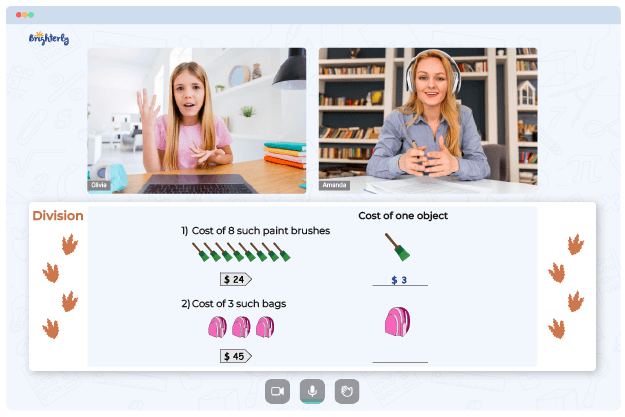# Dividing Decimals by Whole Numbers Worksheets

To divide a decimal, you have to use a whole number as your divisor, and this process is part of the basic arithmetic that kids must learn. However, with decimals, the rules differ. Kids require extra help when learning to divide decimals by whole numbers: they need the dividing decimals by whole numbers worksheets.### Dividing Decimals by Whole Numbers Worksheets PDF

Dividing Whole Numbers By Decimals Worksheet### Dividing Decimals by Whole Numbers Worksheets PDF

Dividing Decimals By Whole Numbers Worksheet### Dividing Decimals by Whole Numbers Worksheets PDF

Divide Decimals By Whole Numbers Worksheet### Dividing Decimals by Whole Numbers Worksheets PDF

Dividing A Decimal By A Whole Number Worksheets

## Benefits of Using Dividing Decimals by Whole Numbers Worksheets

The use of dividing decimals by whole numbers worksheets provides benefits for both teachers and students, and here are some of them.

### Simplifying the teaching process for teachers

Teachers won’t go home with their students daily to ensure kids get the lessons right. So, they need a tool to ensure that students practice challenging topics like decimals. When kids go home and use decimals worksheets, they solve some questions, get some wrong, and learn.

When kids return to class, their tutors can go through the worksheets to see where a student had challenges and then help them improve. Instead of watching each child, teachers can simply use a worksheet as a metric to see how well kids are doing with dividing decimals with whole numbers. In this way, the tool becomes a gauge for understanding students’ level.

Math for Kids

Is Your Child Struggling With Math?
1:1 Online Math Tutoring### Making learning fun for kids

A worksheet is usually filled with fun and interactive practice exercises that kids can use to boost their math skills. Sometimes, students do not enjoy classwork because it is not very interesting.

And worksheets come in colorful designs, and the creators ensure the questions are fun and exciting. When kids find a learning process engaging, they are more inclined to get better at the concept behind that learning process.

### Simplifying the complexity of decimals

Decimals come off as a complex and challenging topic but become easy math concepts with repetition. When a child repeatedly uses a divide decimals by whole numbers worksheet, the decimals seem not so complicated, but much easier. The more time kids spend with worksheets of different complexity, the better they get at dividing decimals with whole numbers.

## Download Dividing Decimals by Whole Numbers Worksheets in Printable PDF Format

If you need help in teaching a child how to get through dividing decimals by a whole number, worksheets with colorful images will come in handy. There are many math platforms on the internet where you can get all kinds of dividing decimals by whole numbers worksheets.

Some teachers would want to go through the process of creating their own worksheets for kids. Educators design worksheets according to the demands of today’s math curriculum, especially for complex math concepts such as decimals. Simply go on a math website and download a printable PDF of dividing decimals by whole numbers worksheets and share them with your kids.

### More Decimals Worksheets

Struggling with Division?• Does your child need extra assistance with mastering division lessons?
• Start learning with an online tutor.

Is your child finding it hard to understand division concepts? An online tutor could be of help.